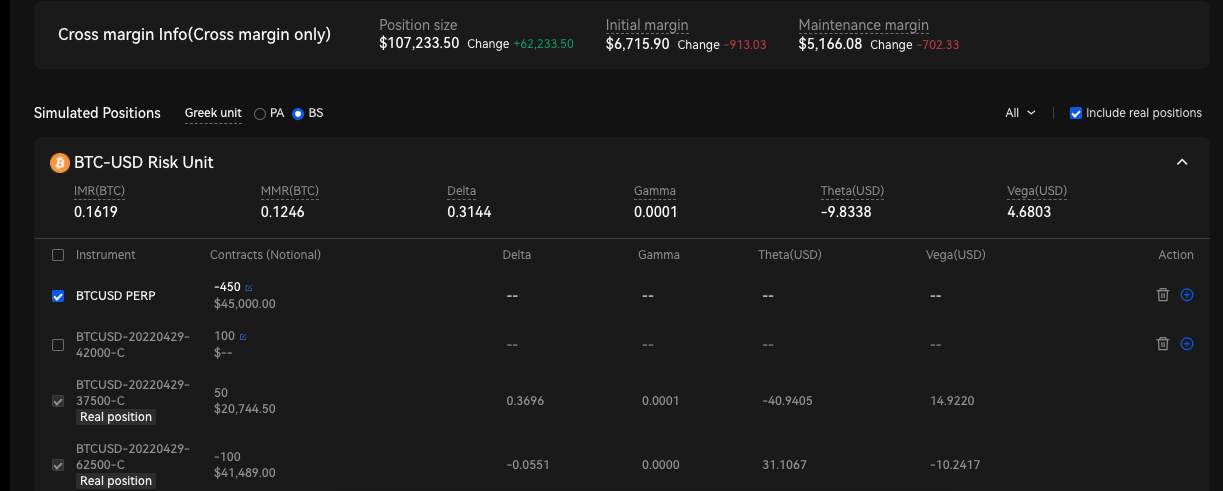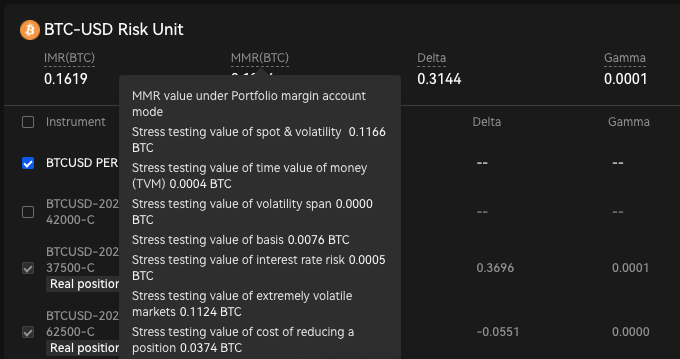# Ⅵ. Multi-currency margin mode vs. Portfolio margin modeOKX

### 1. Introduction

As a leading derivatives exchange, OKX strives to provide our users with the best-in-class margin infrastructure. To further enhance how users utilize capital, the OKX unified account has iterated from Single-, Multi-currency margin to the latest Portfolio margin.

For details on multi-currency margin and portfolio margin, refer to the following:

Ⅳ. Multi-currency margin mode

V. Portfolio margin mode

Key Concepts

Terms

Definition

Basic concepts（the same applies to portfolio margin mode）

Balance

Cash balance of each currency

Equity

Balance + Unrealized PNL + Option Market Value - Accrued Interest

UPL（Unrealized P&L）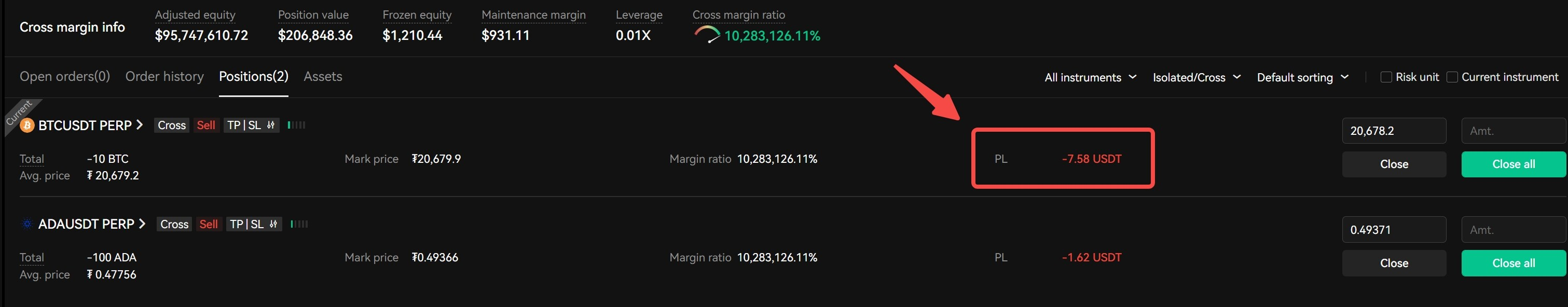The current P&Ls from a specific position that has not been realized (squared)

Margin Mode

Isolated: limited risk with segregated position and margin

Cross: greater capital efficiency with PnL offset

Cross Margin Ratio

Adjusted Equity / (MMR + liquidation cost)

Initial Margin Requirement (IMR)

The margin value required to open new orders

Maintenance Margin Requirement (MMR)(non-PM mode)

The margin value required to maintain current positions, to evaluate whether to go to liquidation process

Portfolio margin mode key Concepts

Maintenance Margin Requirement (MMR)(only PM mode)

Maintenance Margin Requirement (MMR) is determined in risk unit basis, where all instruments (perpetual swaps, futures, options, and spot under the spot-derivatives risk offset mode) are grouped by underlyings to simulate the maximum loss that can occur in a portfolio under a specific set of market conditions. The USD-value of the individual MMRs will then be summed up into a portfolio MMR (in USD value)

Portfolio margin consists of derivatives margin and borrowing margin. Portfolio margin calculates sums up derivative margin under each risk unit and then adds borrowing margin to obtain the margin at portfolio level.

MMR = Sum of USD value of each risk unit Derivatives MMR+ account level Borrowing MMR = max { [max(spot shock, theta decay risk, extreme move) + basis risk + vega risk + interest rate risk], adjusted minimum charge}

Risk factor(MR)

Derivatives margin calculates 6 risks (MR1-6) by stress testing the portfolio under a specific set of market conditions of each risk unit, and then applying the minimum charge (MR7). Minimum charge is designed to cover any liquidation fee, transaction cost, and slippage.

Risk Unit

All derivatives are grouped into risk units based on their underlyings (e.g. BTC-USD, BTC-USDT, ETH-USD, ETH-USDT, etc).

The perpetual swaps, futures, and options with the same underlying are considered holistically within the risk unit. Margins are calculated per risk-unit, so as to factor in risk-offsetting among instruments.

Spot in use

Under spot-derivatives risk offset mode, spot in use, or how much spot is used under the risk unit is determined by the delta of derivatives that belong to the same risk unit.

Derivatives type

Under spot-derivatives risk offset mode, the user could choose to place spot in either USDT or crypto-margined risk unit.

*Spot-derivatives (USDT) risk offset mode: the underlying spot is placed into USDT-margined risk unit.

**Spot-derivatives (Crypto) risk offset mode: the underlying spot is placed into crypto-margined risk unit.

Scenario-based Margin calculation

Comparing with Unified account, we adopt a more scientific and rigorous risk model: maintenance margin is calculated from stress test values in 8 dimensions. Customers with large positions or doing risk hedging can enjoy considerable discounts on their margin requirements.

Mode

SOL-USDT Risk Unit

SOL-USD Risk Unit

Derivatives-only

SOL-USDT Perpetual swaps, futures

SOL-USD perpetual swaps, futures, options

Spot-derivatives (USDT)

SOL-USDT Perpetual swaps, futures & SOL

SOL-USD Perpetual swaps, futures, options,

Spot-derivatives (coin)

SOL-USDT Perpetual swaps, futures

SOL-USD Perpetual swaps, futures, options & SOL

### 2. Comparison of Multi-currency Margin Mode and Portfolio Margin Mode

Account mode

Multi-currency margin（leverage:5x）

Portfolio margin 1.0

(Derivatives-only mode)

（leverage:5x）

Portfolio margin 2.0

(Spot-derivatives risk offset mode)

（leverage:5x）

All instruments (spot, margin, perpetual swap, futures, and options)

Prerequisite

Equity > 10K USD

Equity > 10K USD

Applicable collaterals

All assets in trading accounts can be used as collateral, by valuing them in USD equity upon a tier-based discounting. (Discount rate)

Unrealized P&Ls in derivatives positions can be used as equity in corresponding assets (known as P&L offset).

Treatment of option value

Long option positions are placed in isolated mode. Only short option positions are considered as available margin in cross-margin mode.

Both long and short options can be evaluated in cross-margin mode; therefore, the values of both are considered as available margin in cross-margin mode.

Position margining

Positions in different instruments are independently margined based on position tiers. (Position tiers)

Derivatives positions are grouped by risk-unit. Their risks are assessed holistically under different scenarios (V. Portfolio Margin mode), and the required margin is calculated based on the maximum loss in all scenarios.

Derivatives positions are grouped by risk-unit. Their risks are assessed holistically under different scenarios (V. Portfolio Margin mode), and the required margin is calculated based on the maximum loss in all scenarios.

Additionally, the delta position from spot assets can be included in the corresponding risk units (either USDT-margined or crypto-margined).

e.g., BTC spot assets in an account can be included in a BTC-USD or BTC-USDT risk unit for delta risk offsetting.

### 3. Example of Multi-currency Margin Mode vs. Portfolio Margin Mode

Assets

148 ETH

Mode\Positions

-30000 ETH-USDT-SWAP

20000 ETH-USDT-0930

Multi-currency margin

MMR = 7,947 USD

Portfolio margin 1.0 (only-derivatives risk offset) margin

MMR = 33,665 USD

Porfolio margin 2.0 (spot-derivatives risk offset) margin

MMR = 9,618 USD (spot in use = 100 ETH)

Optimization on MMR (Portfolio margin 1.0 ——> Portfolio margin 2.0）

Save 70%

Conclusion

In portfolio margin mode, users with larger assets, offsetting position structures, and spot positions will receive a smaller MMR and more flexible funds.

In some scenarios, Portfolio margin 2.0 MMR is better than multi-currency margin MMR, which optimizes MMR calculation in small holdings.

### 4. Exploration Tools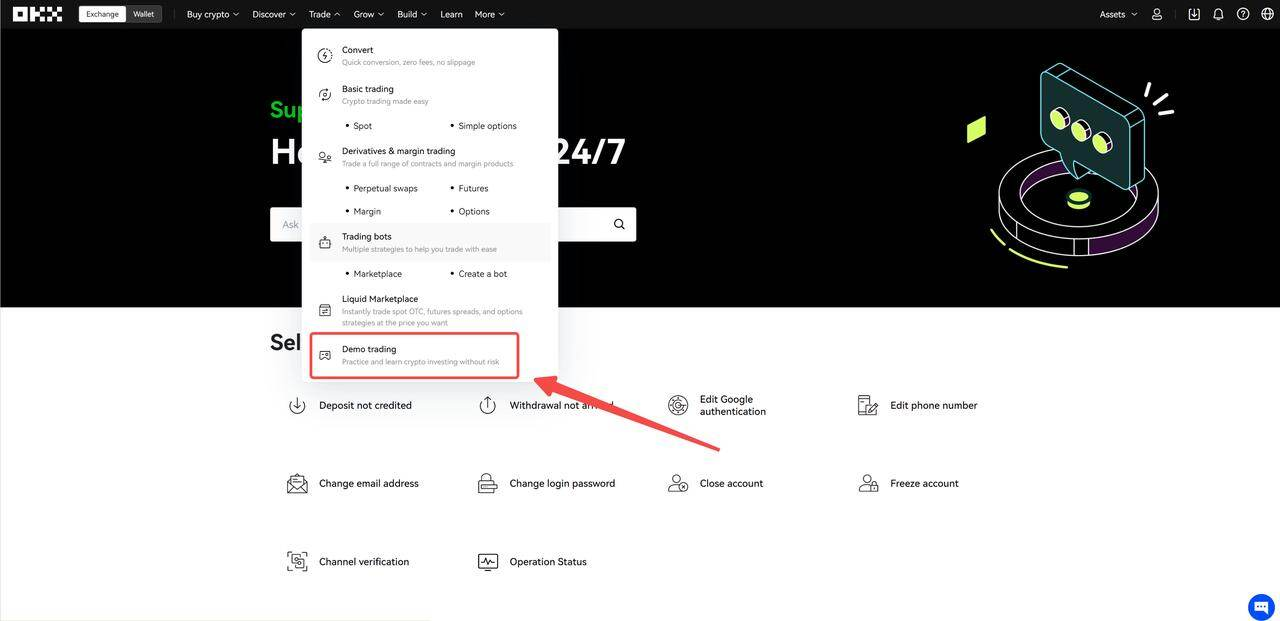Cross margin mode

Trade------Settings------Account mode------Cross-margin mode (Net Equity > 50K)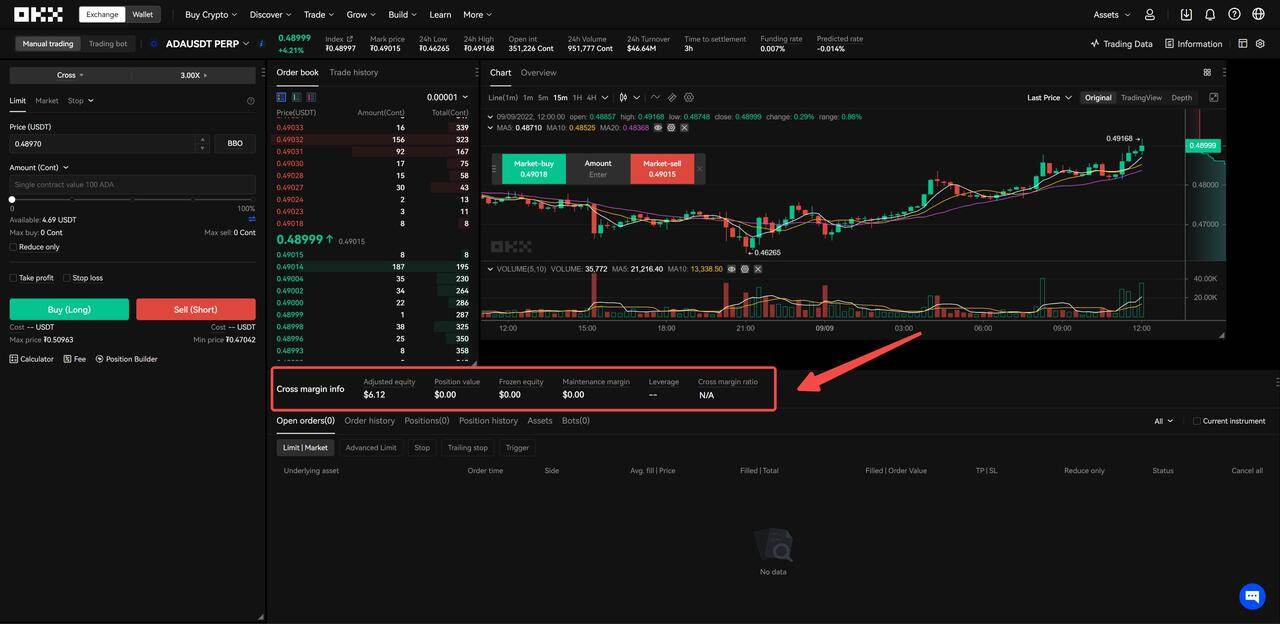For details on the equity, MMR, and margin ratio in cross-margin mode, refer to the following:

Ⅳ. Multi-currency margin mode: cross margin trading

Portfolio margin mode

Trade------Settings------Account mode------ Portfolio margin (Net Equity > 100K)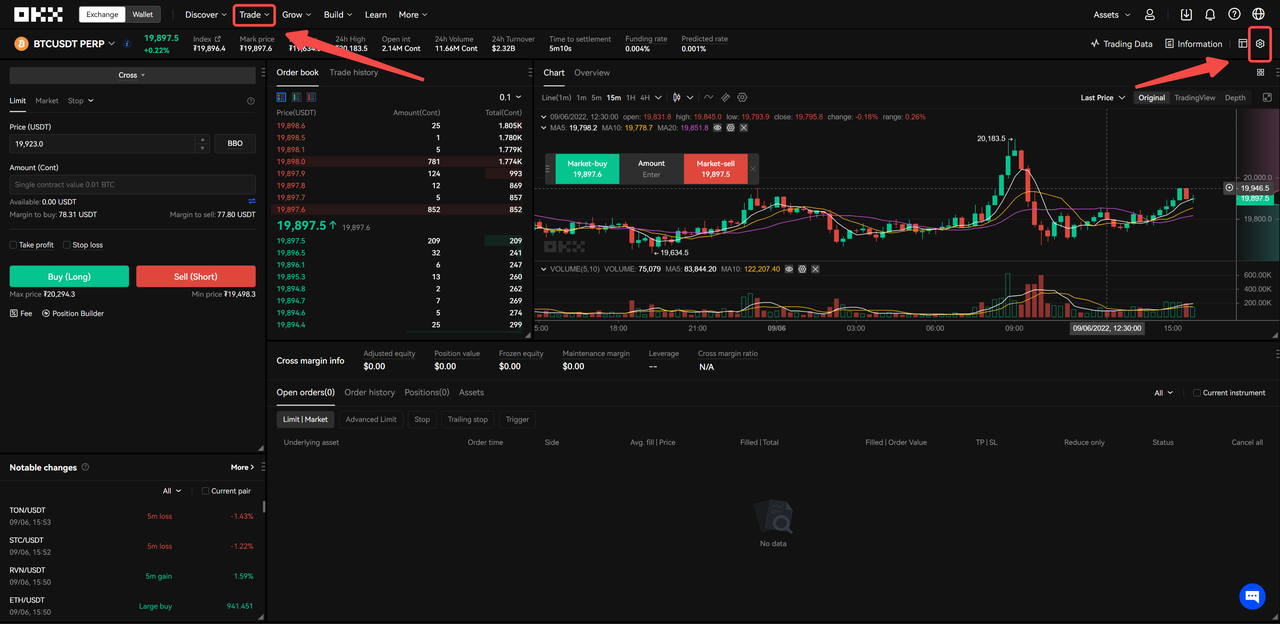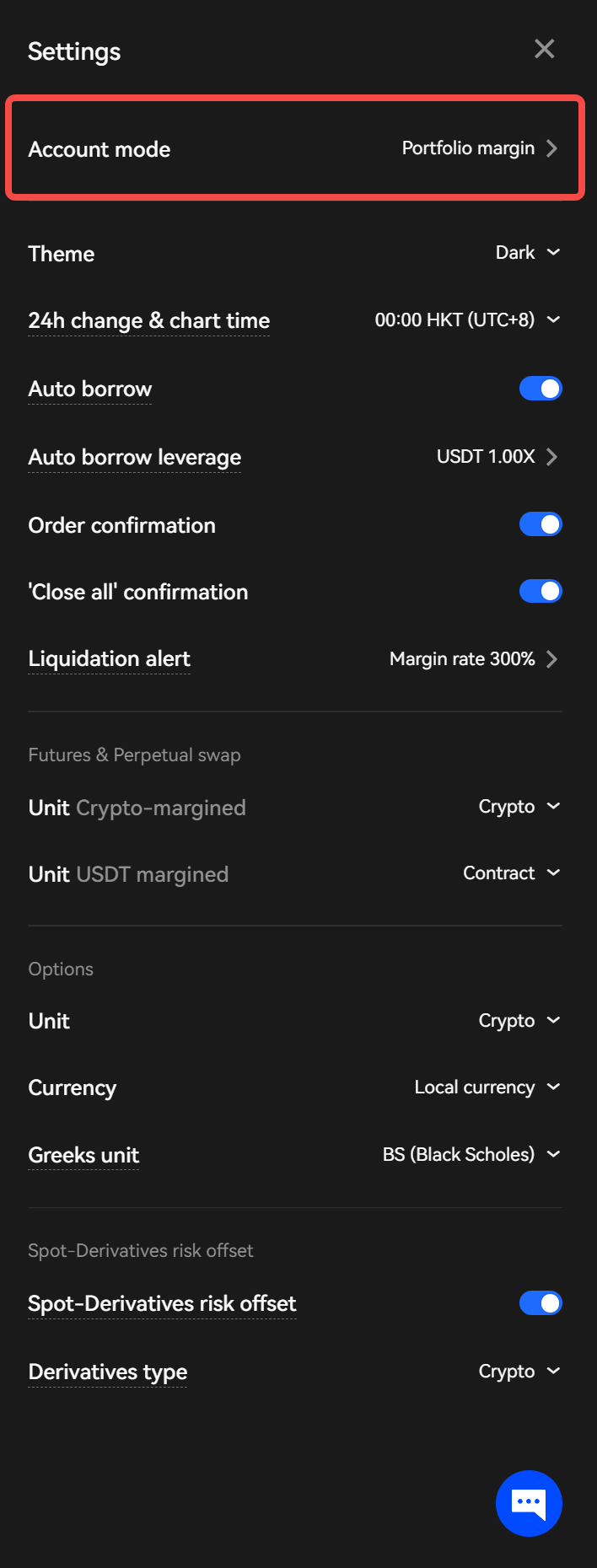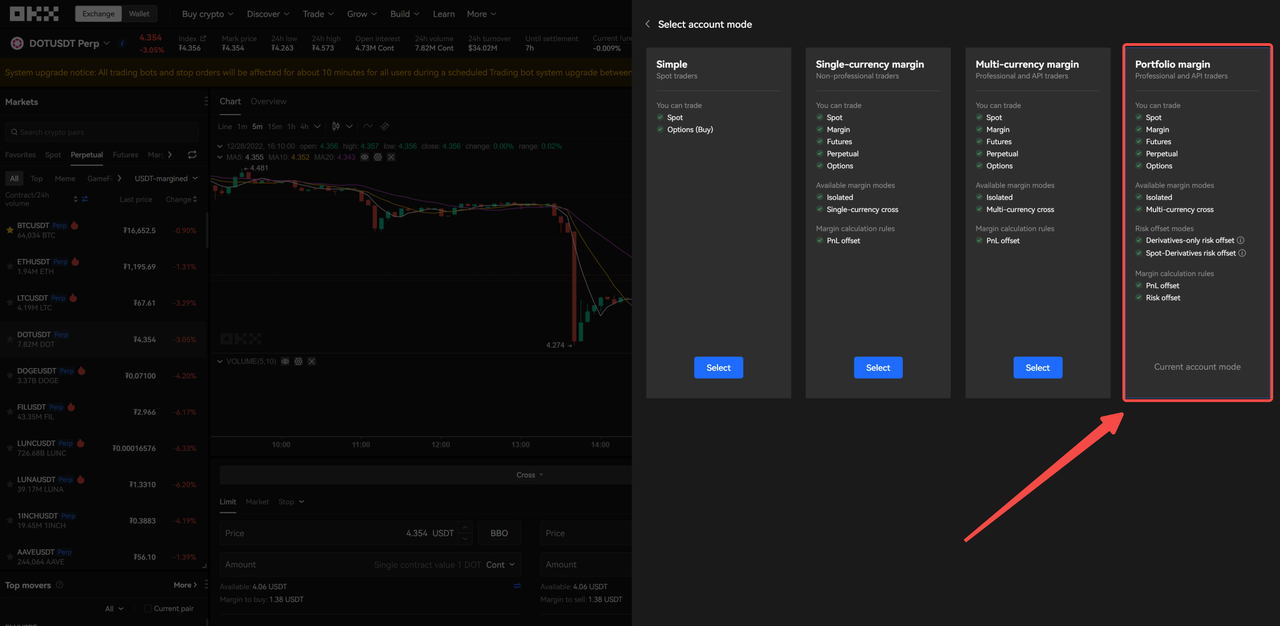Choose whether to turn on/off the spot-derivatives risk offsets button --- observe your spot hedging quantity on the positions and assets page (the first time you enter, the default "derivatives type" is USDT mode):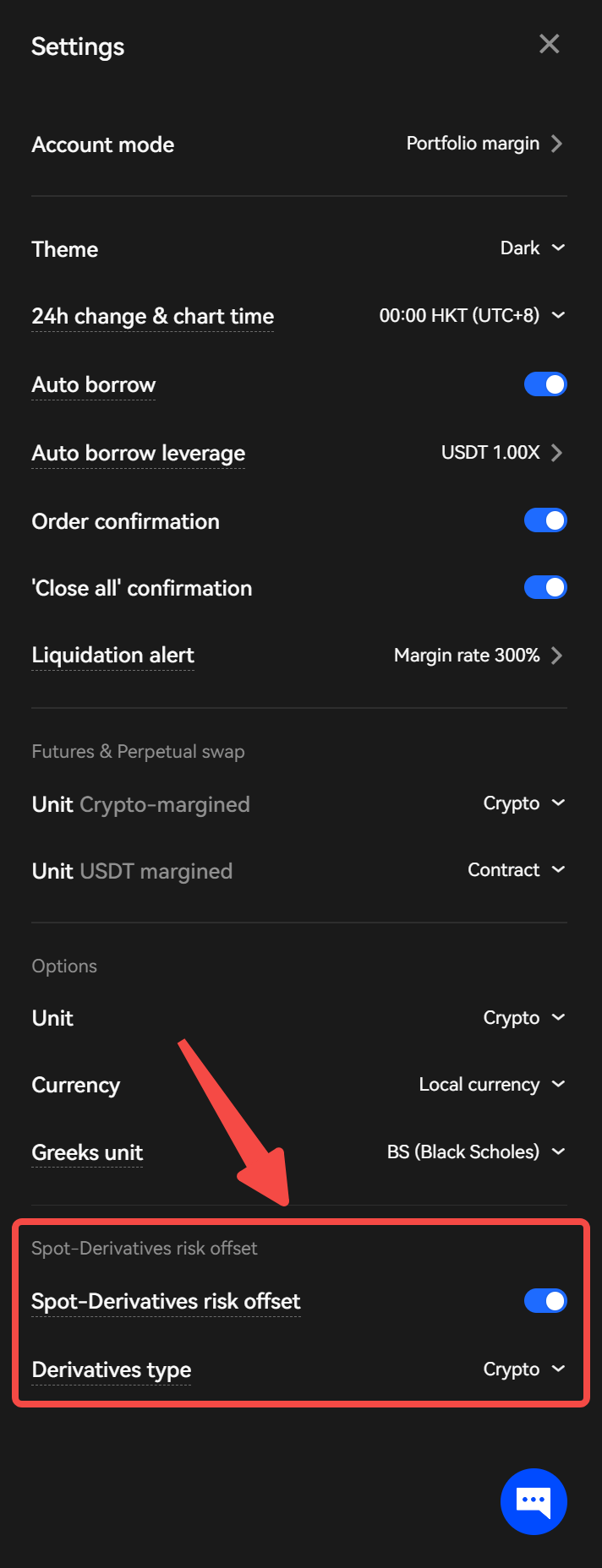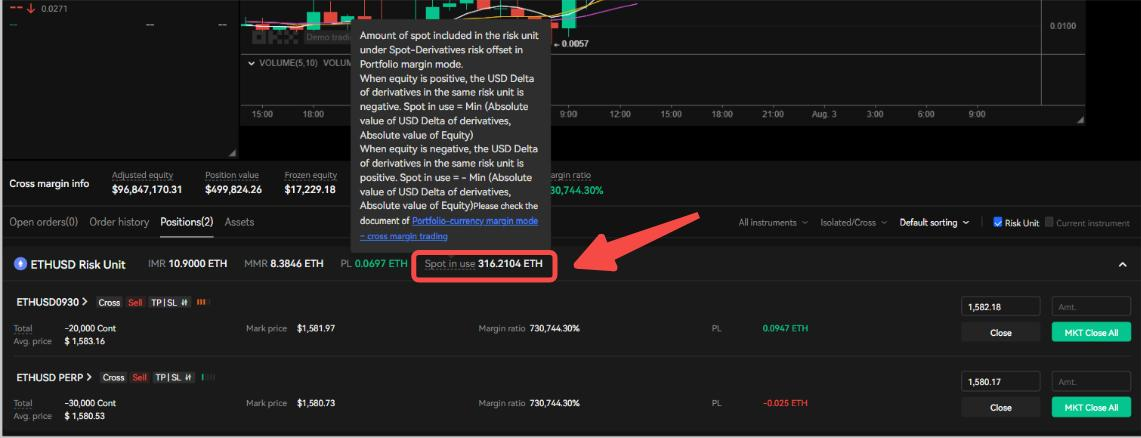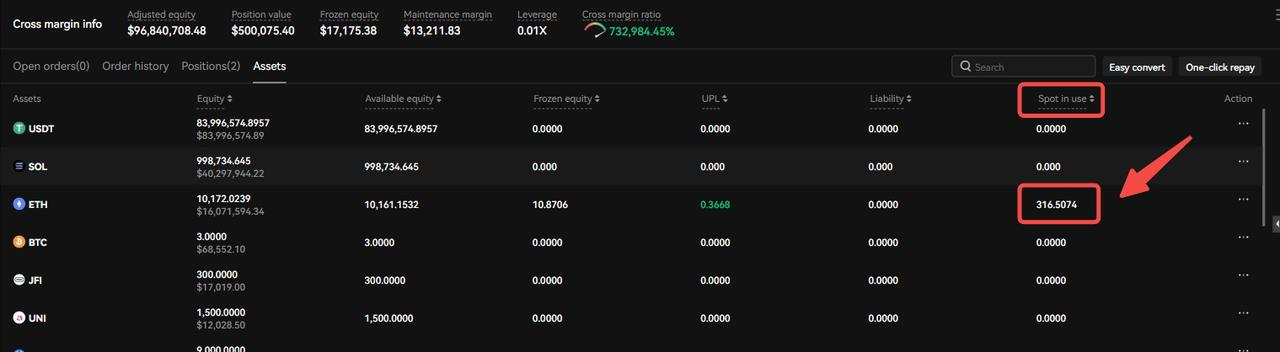For details on the equity, MMR, and margin ratio in portfolio margin mode, refer to the following:

V. Portfolio Margin mode: cross margin trading

#### 2. Position builder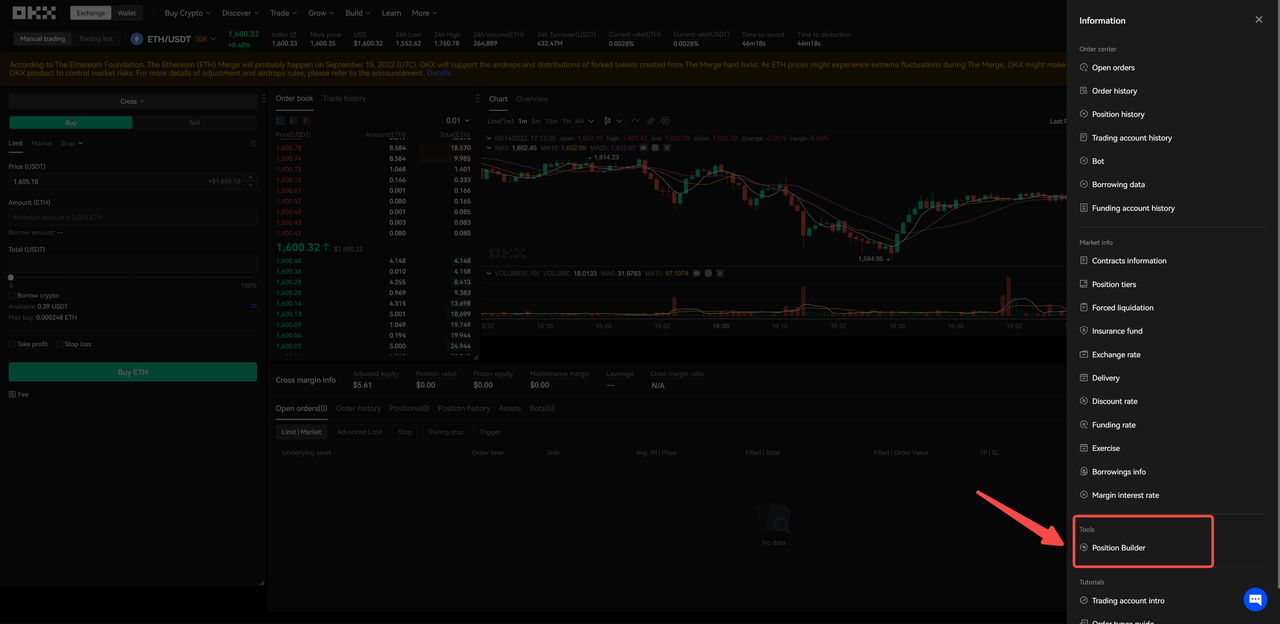You can simulate new positions in position builder to see the IMR and MMR, as shown below. Additionally, you can include existing positions with simulated positions to simulate the impact on IMR and MMR: